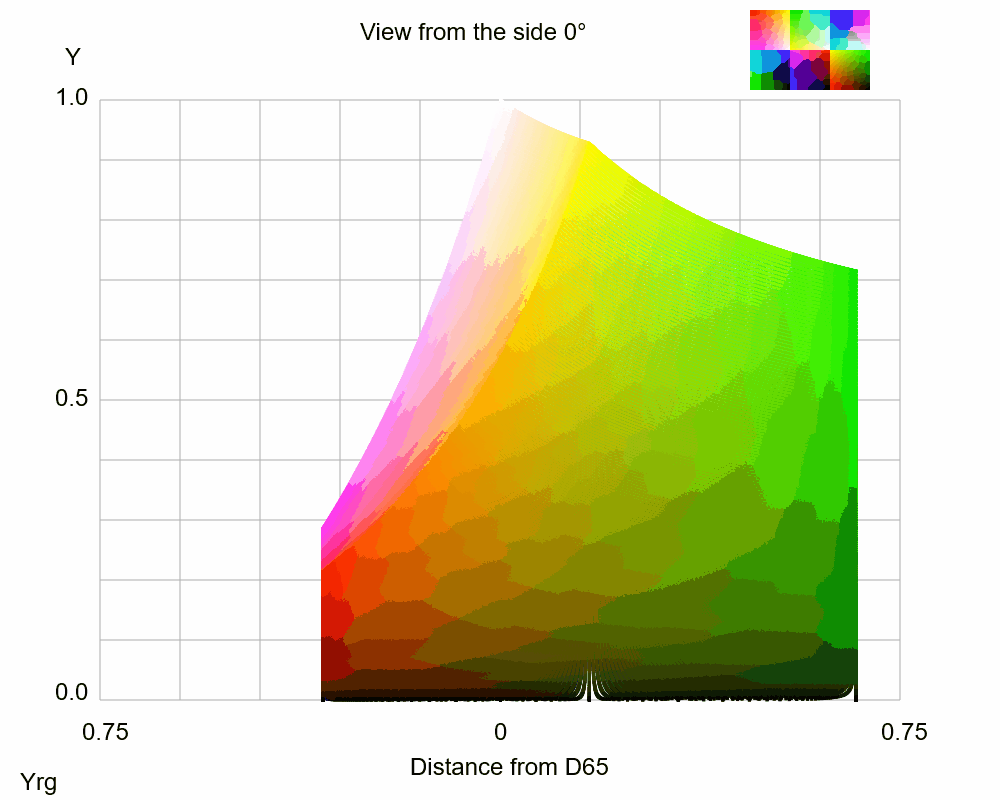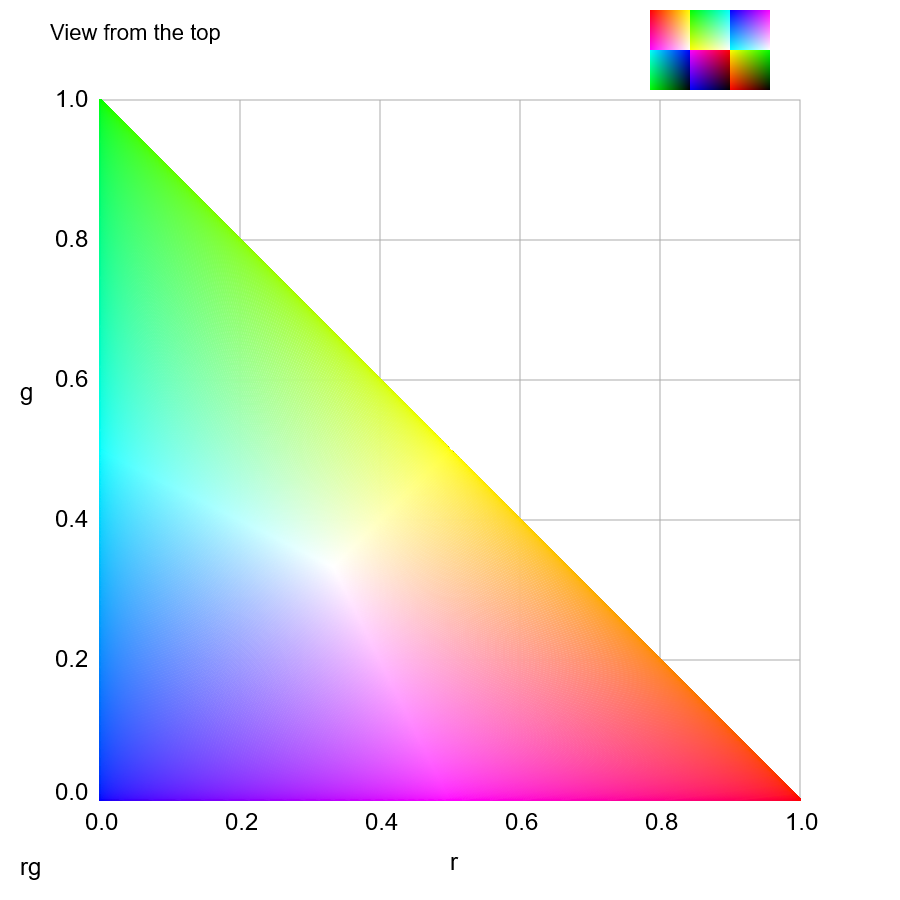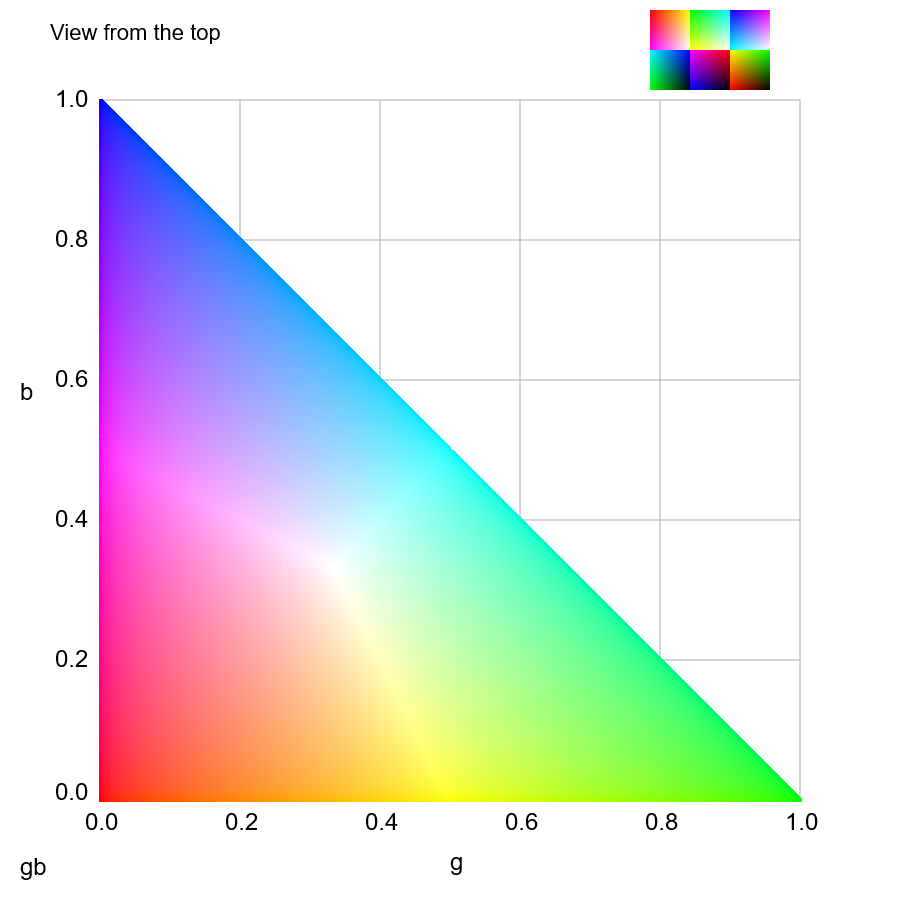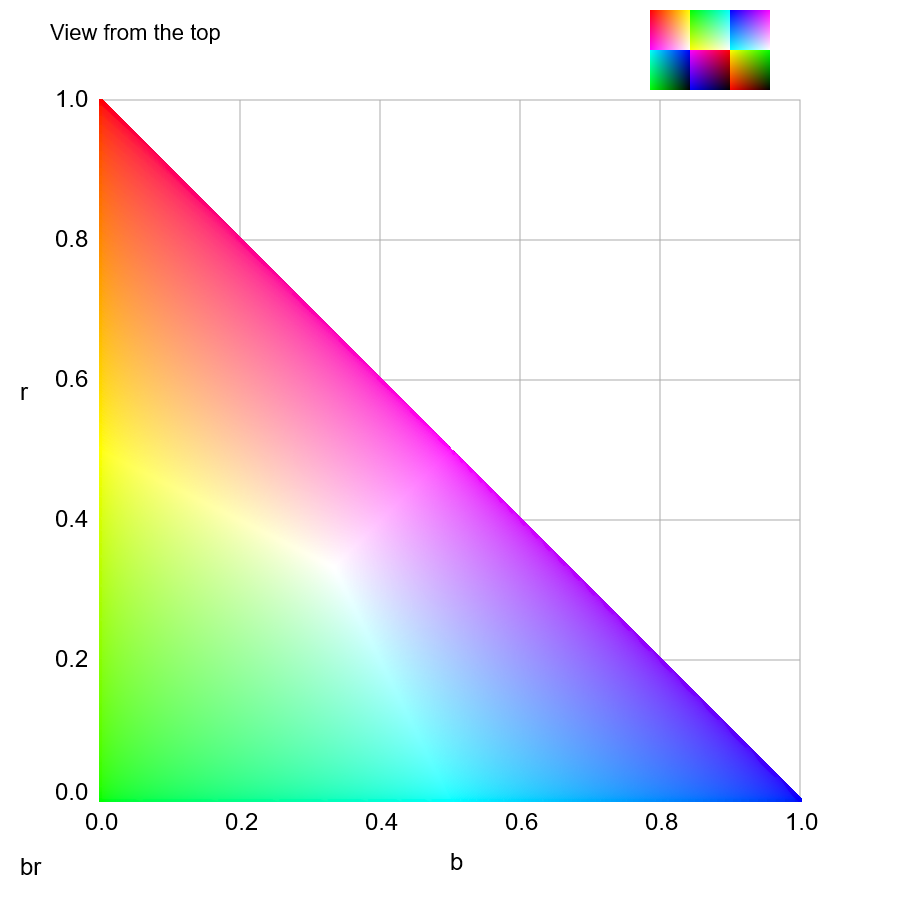Yrg color space

since June 5, 2023r = R_linear / (R_linear + G_linear + B_linear)
g = R_linear / (R_linear + G_linear + B_linear)
b = B_linear / (R_linear + G_linear + B_linear)Top viewJavaScript programs

Akiyoshi's illusion pages